# S 8 P 3 Students will investigate relationship

• Slides: 33
Download presentationS 8 P 3. Students will investigate relationship between force, mass, and the motion of objects. c. Demonstrate the effect of simple machines (lever, inclined plane, pulley, wedge, screw, and wheel and axle) on work.1. Define machine, simple machine, effort force, resistance force, and mechanical advantage. . 2. Explain how mechanical advantage changes the input force. 3. List and describe the characteristics of the six simple machines. 4. Cite everyday examples of each type of simple machine.What price do you pay to have a machine make your work easier?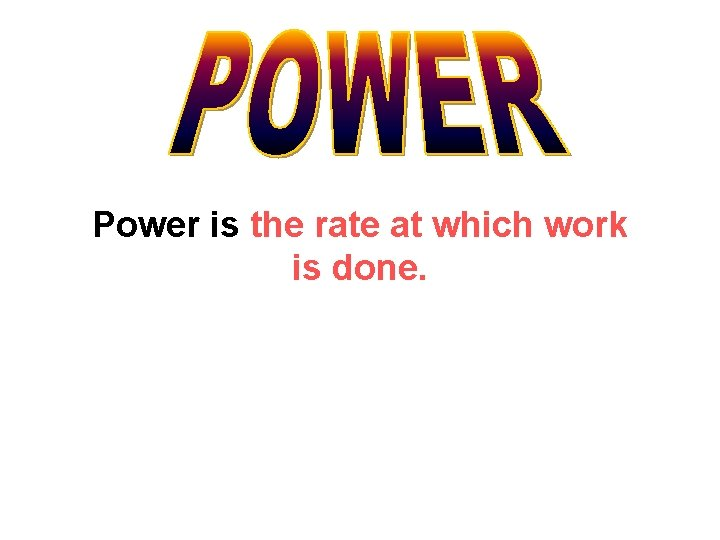Power is the rate at which work is done.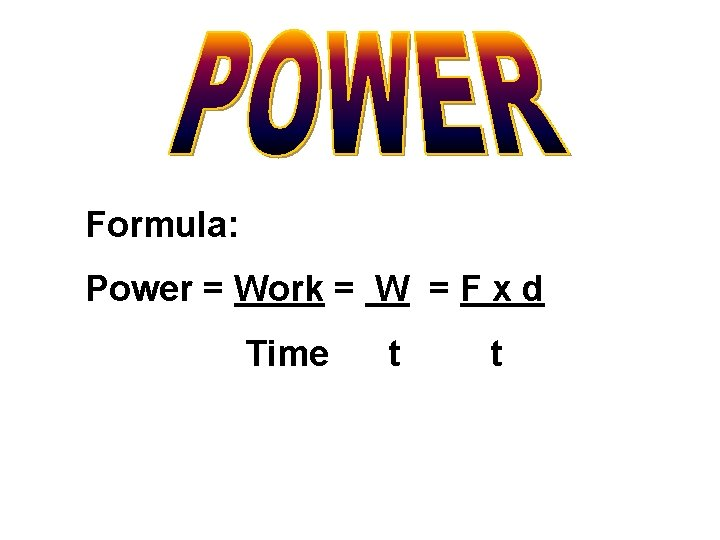Formula: Power = Work = W = F x d Time t t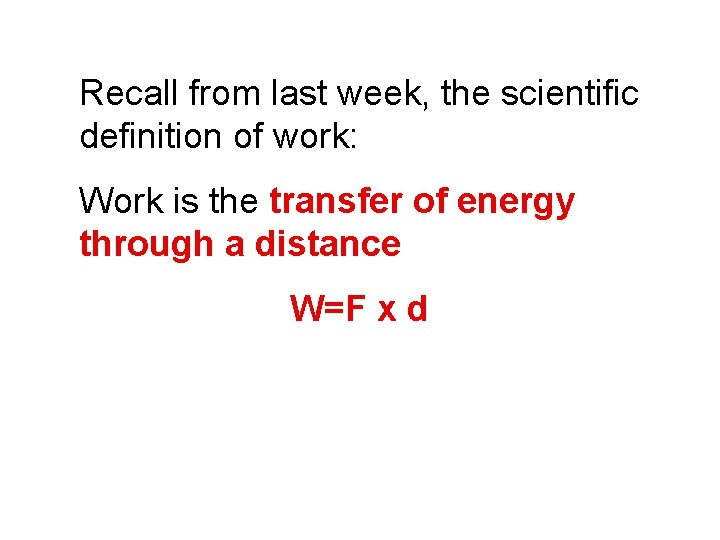Recall from last week, the scientific definition of work: Work is the transfer of energy through a distance W=F x dExample #3 How much power does a person weighing 500 N need to climb a 3 -m ladder in 5 seconds?Power is measured in watts, named for James Watt, who worked on the steam engine One watt = one joule per secondA watt is pretty small – about equal to the power used to raise a glass of water from your knees to your mouth in one second.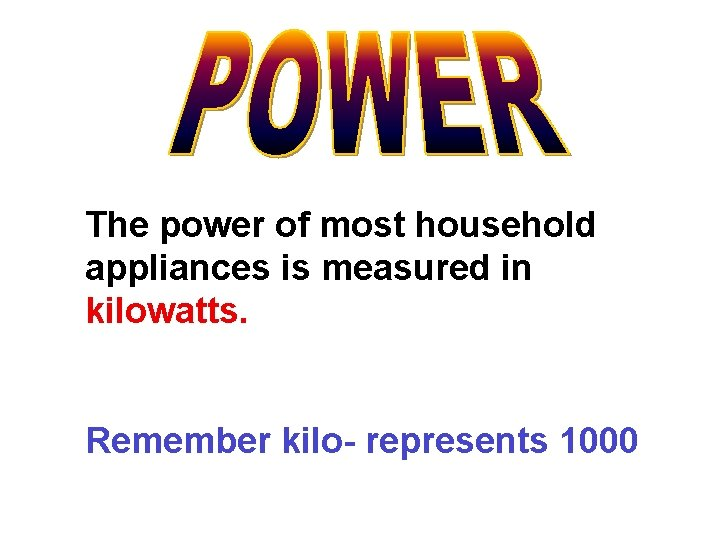The power of most household appliances is measured in kilowatts. Remember kilo- represents 1000Historically, power was provided by animals (i. e. horses)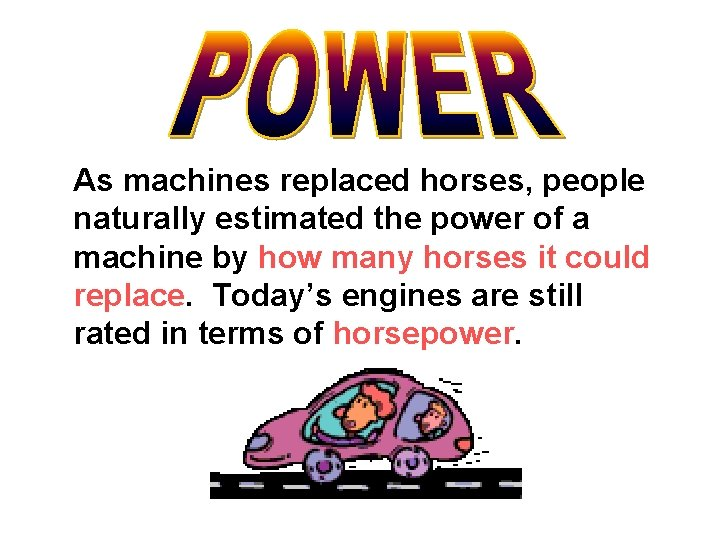As machines replaced horses, people naturally estimated the power of a machine by how many horses it could replace. Today’s engines are still rated in terms of horsepower.REMEMBER: Energy: Ability to do work Work Force x Distance = Force: A Push or a PullA machine is a device that makes your task easier! In general, machines allow you to overcome gravity, inertia, and/or friction in order to move something!A machine is any device that can change the strength of a force, its speed, or its direction, but a machine does not reduce the amount of work to be done.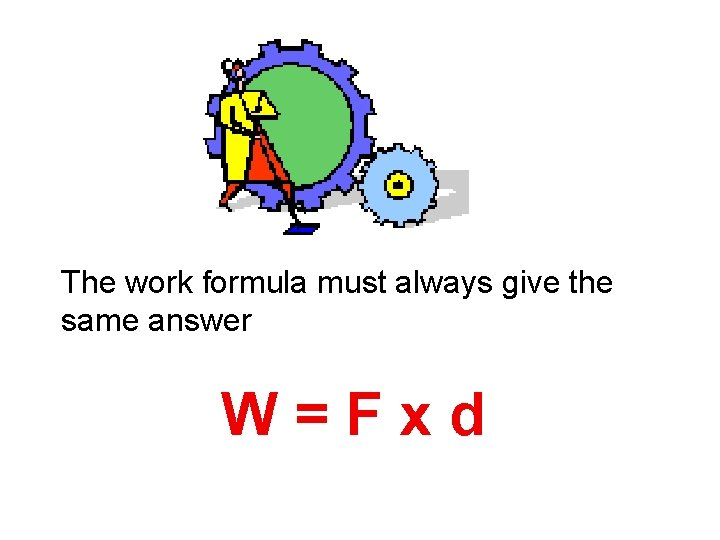The work formula must always give the same answer W=Fxd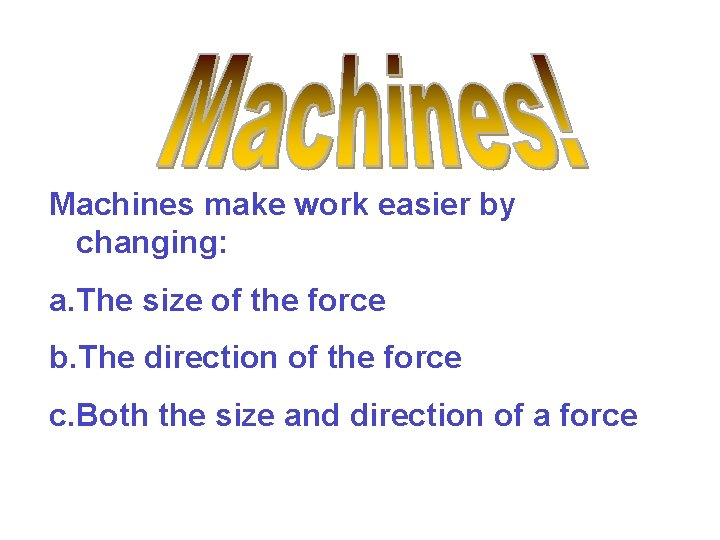Machines make work easier by changing: a. The size of the force b. The direction of the force c. Both the size and direction of a force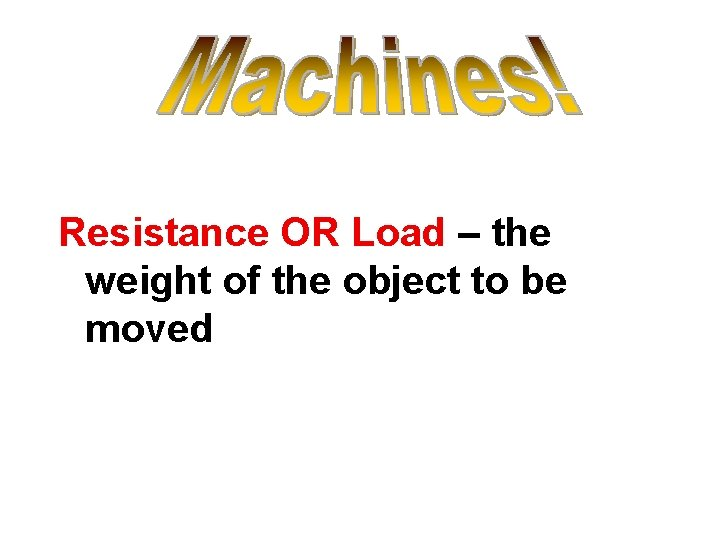Resistance OR Load – the weight of the object to be moved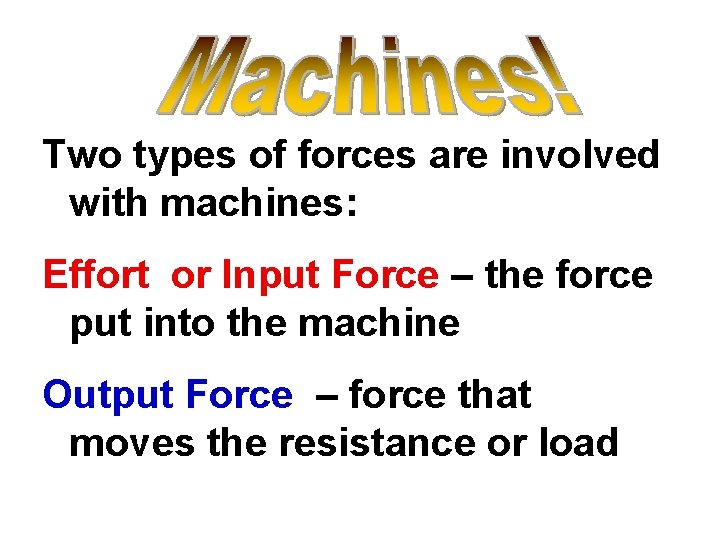Two types of forces are involved with machines: Effort or Input Force – the force put into the machine Output Force – force that moves the resistance or load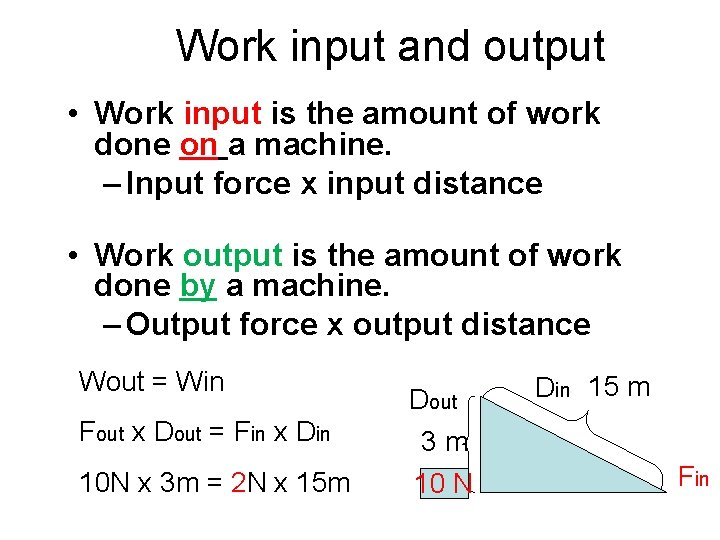Work input and output • Work input is the amount of work done on a machine. – Input force x input distance • Work output is the amount of work done by a machine. – Output force x output distance Wout = Win Fout x Dout = Fin x Din Dout 3 m 10 N x 3 m = 2 N x 15 m 10 N Din 15 m Fin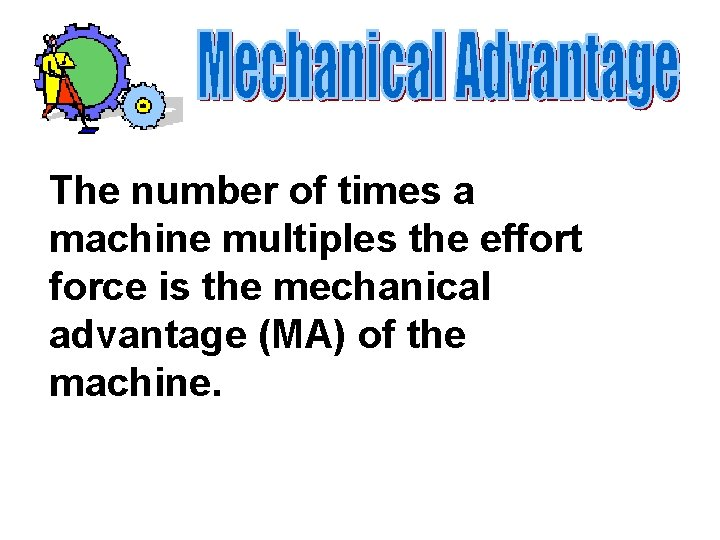The number of times a machine multiples the effort force is the mechanical advantage (MA) of the machine.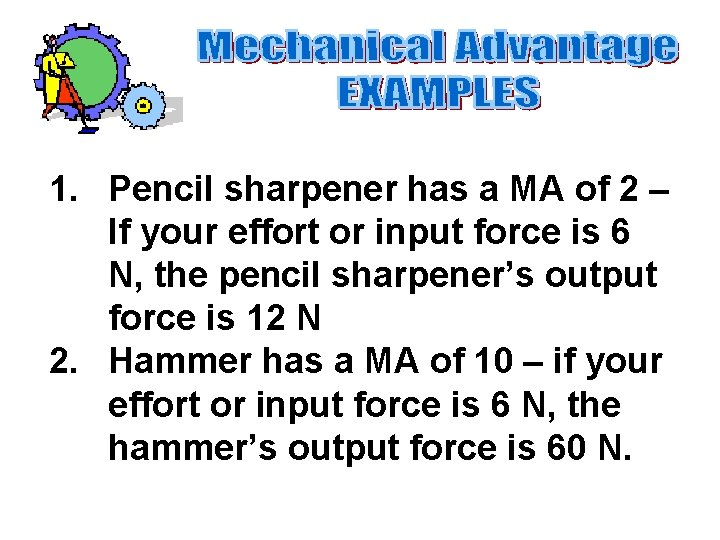1. Pencil sharpener has a MA of 2 – If your effort or input force is 6 N, the pencil sharpener’s output force is 12 N 2. Hammer has a MA of 10 – if your effort or input force is 6 N, the hammer’s output force is 60 N.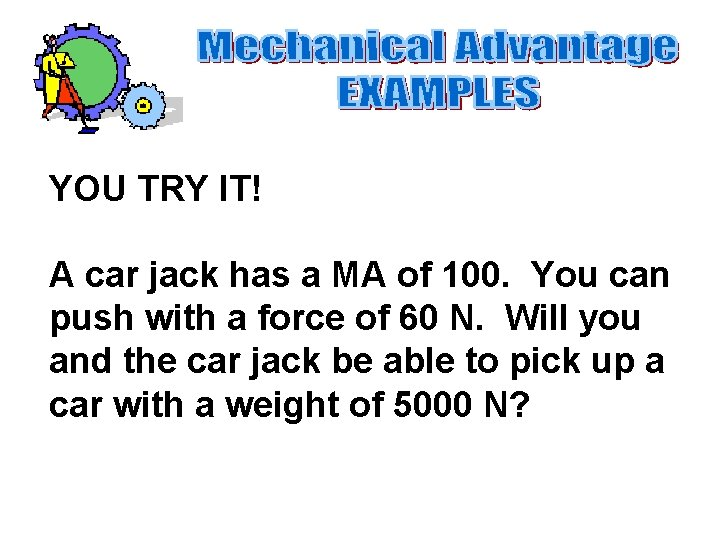YOU TRY IT! A car jack has a MA of 100. You can push with a force of 60 N. Will you and the car jack be able to pick up a car with a weight of 5000 N?Different machines have different mechanical advantages. Remember no matter what the mechanical advantage is, the amount of work is the same!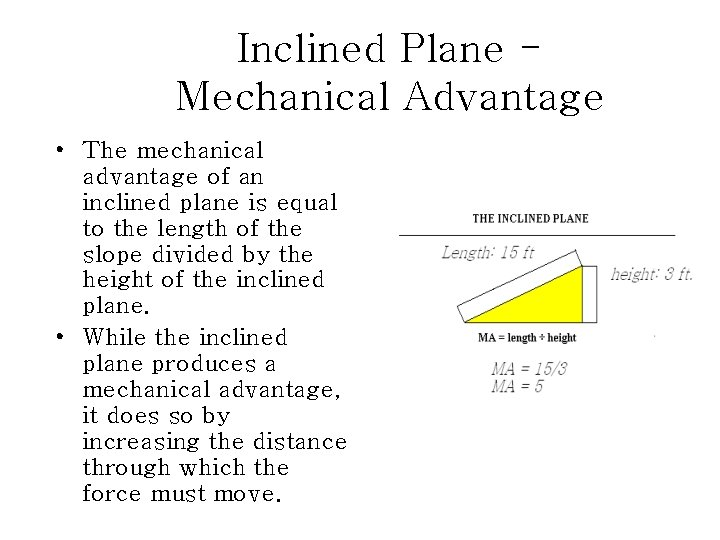Inclined Plane Mechanical Advantage • The mechanical advantage of an inclined plane is equal to the length of the slope divided by the height of the inclined plane. • While the inclined plane produces a mechanical advantage, it does so by increasing the distance through which the force must move.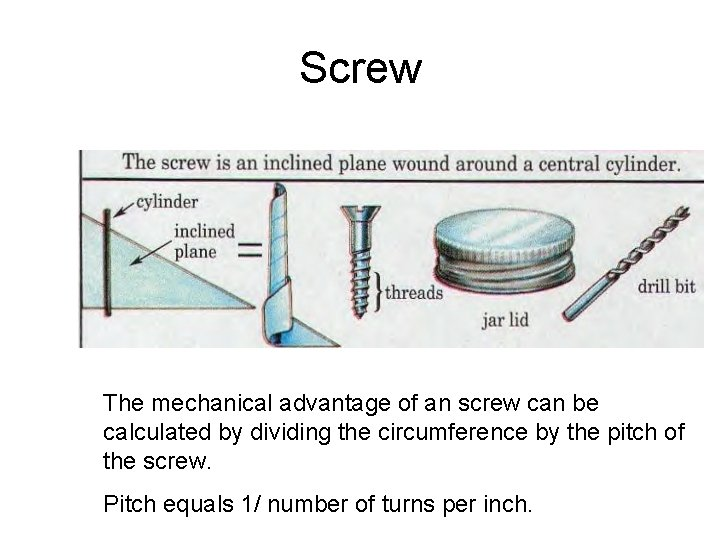Screw The mechanical advantage of an screw can be calculated by dividing the circumference by the pitch of the screw. Pitch equals 1/ number of turns per inch.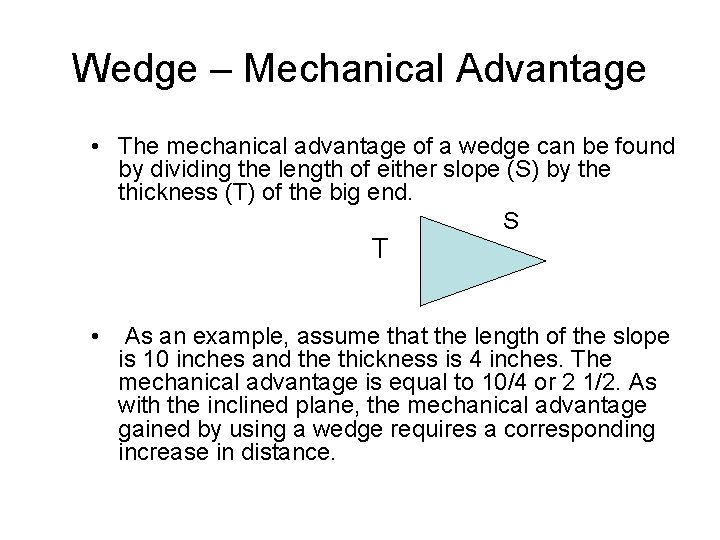Wedge – Mechanical Advantage • The mechanical advantage of a wedge can be found by dividing the length of either slope (S) by the thickness (T) of the big end. S T • As an example, assume that the length of the slope is 10 inches and the thickness is 4 inches. The mechanical advantage is equal to 10/4 or 2 1/2. As with the inclined plane, the mechanical advantage gained by using a wedge requires a corresponding increase in distance.Fulcrum is between EF (effort) and RF (load) Effort moves farther than Resistance. Multiplies EF and changes its direction The mechanical advantage of a lever is the ratio of the length of the lever on the applied force side of the fulcrum to the length of the lever on the resistance force side of the fulcrumDiagrams of Pulleys Fixed pulley: Movable Pulley: A fixed pulley changes the direction of a force; however, it does not create a mechanical advantage. The mechanical advantage of a moveable pulley is equal to the number of ropes that support the moveable pulley.COMBINED PULLEY • The effort needed to lift the load is less than half the weight of the load. • The main disadvantage is it travels a very long distance.Wheel and Axel • The mechanical advantage of a wheel and axle is the ratio of the radius of the wheel to the radius of the axle. 1 5 • In the wheel and axle illustrated above, the radius of the wheel is five times larger than the radius of the axle. Therefore, the mechanical advantage is 5: 1 or 5. • The wheel and axle can also increase speed by applying the input force to the axle rather than a wheel. This increase is computed like mechanical advantage. This combination would increase the speed 5 times.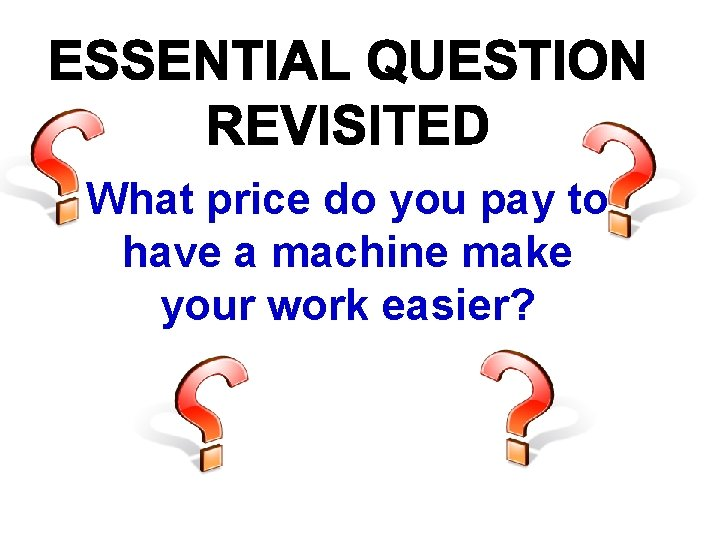What price do you pay to have a machine make your work easier?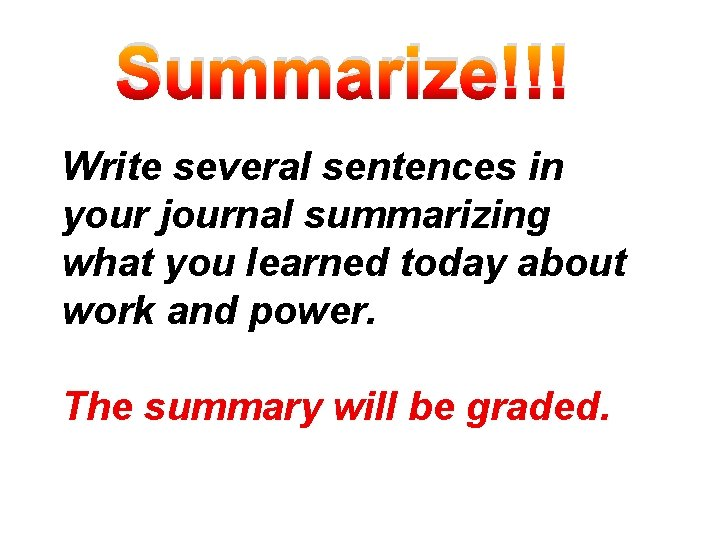Summarize!!! Write several sentences in your journal summarizing what you learned today about work and power. The summary will be graded.